# Electric circuits – problems and solutions

1. R1, = 6 Ω, R2 = R3 = 2 Ω, and voltage = 14 volt, determine the electric current in circuit as shown in figure below.

Known :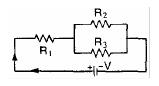Resistor 1 (R1) = 6 Ω

Resistor 2 (R2) = 2 Ω

Resistor 3 (R3) = 2 Ω

Voltage (V) = 14 Volt

Wanted : Electric current (I)

Solution :

Equivalent resistor (R) :

R2 and R3 are connected in parallel. The equivalent resistor :

1/R23 = 1/R2 + 1/R3 = 1/2 + 1/2 = 2/2

R23 = 2/2 = 1 Ω

R1 and R23 are connected in series. The equivalent resistor :

R = R1 + R23 = 6 Ω + 1 Ω

R = 7 Ω

Electric current (I) :

I = V / R = 14 / 7 = 2 Ampere

[irp]

2. Which one of the electric circuits as shown below has the bigger current.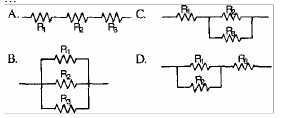Solution :

The resistance of the resistor is R and the electric voltage is V.

R1, R2 and R3 are connected in series. The equivalent resistor :

RA = R1 + R2 + R3 = R + R + R = 3R

Electric current (I) :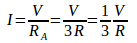R1, R2 and R3 are connected in parallel. The equivalent resistor :

1/R = 1/R1 + 1/R2 + 1/R3 = 1/R + 1/R + 1/R = 3/R

RB = R/3

Electric current (I) :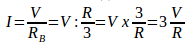R2 and R3 are connected in parallel. The equivalent resistor :

1/R23 = 1/R2 + 1/R3 = 1/R + 1/R = 2/R

R23 = R/2

R1 and R23 are connected in series. The equivalent resistor :

RC = R1 + R23 = R + R/2 = 2R/2 + R/2 = 3R/2

Electric current (I) :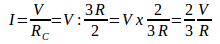R1 and R2 are connected in parallel. The equivalent resistor :

1/R12 = 1/R1 + 1/R2 = 1/R + 1/R = 2/R

R12 = R/2

R12 and R3 are connected in series. The equivalent resistor :

RD = R12 + R3 = R/2 + R = R/2 + 2R/2 = 3R/2

Electric current (I) :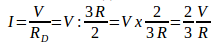[irp]

3. R1 = 4 ohm, R2 = 6 ohm, R3 = 2 ohm, and V = 24 volt. What is the electric current in circuit as shown in figure below.

Known :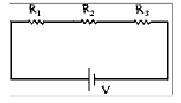Resistor 1 (R1) = 4 Ohm

Resistor 2 (R2) = 6 Ohm

Resistor 3 (R3) = 2 Ohm

Voltage (V) = 24 Volt

Wanted : Electric current in circuit

Solution :

R1, R2 and R3 are connected in series. The equivalent resistor :

R = R1 + R2 + R3 = 4 + 6 + 2

R = 12 Ohm

Electric current :

I = V / R = 24 / 12 = 2 Ampere

[irp]

4. Which one of the electric circuits as shown below has the bigger current.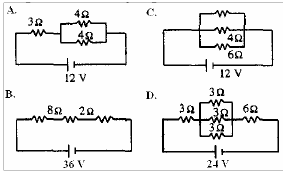Solution

Electric current in circuit A.

The equivalent resistor :

R1 = 3 Ω, R2 = 4 Ω, R3 = 4 Ω, V = 12 Volt

R2 and R3 are connected in parallel. The equivalent resistor :

1/R23 = 1/R2 + 1/R3 = 1/4 + 1/4 = 2/4 = 1/2

R23 = 2/1 = 2 Ω

R1 and R23 are connected in series. The equivalent resistor :

R = R1 + R23 = 3 Ω + 2 Ω = 5 Ω

Electric current (I) :

I = V / R = 12 / 5 = 2.4 Ampere

Electric current in circuit B.

The equivalent resistor :

R1 = 8 Ω, R2 = 2 Ω, R3 = 2 Ω, V = 36 Volt

R1, R2 and R3 are connected in series. The equivalent resistor :

R = R1 + R2 + R3 = 8 + 2 + 2 = 12 Ω

Electric current (I) :

I = V / R = 36 / 12 = 3 Ampere

Electric current in circuit C.

The equivalent resistor :

R1 = 4 Ω, R2 = 4 Ω, R3 = 6 Ω, V = 12 Volt

R2 and R3 are connected in parallel. The equivalent resistor :

1/R23 = 1/R2 + 1/R3 = 1/4 + 1/4 + 1/6 = 3/12 + 3/12 + 2/12 = 8/12

R23 = 12/8 = 1.5 Ω

Electric current (I) :

I = V / R = 12 / 1.5 = 8 Ampere

Electric current in circuit D.

The equivalent resistor :

R1 = 3 Ω, R2 = 3 Ω, R3 = 3 Ω, R4 = 3 Ω, R5 = 6 Ω, V = 24 Volt

R2, R3 and R4 are connected in parallel. The equivalent resistor :

1/R234 = 1/R2 + 1/R3 + 1/R4 = 1/3 + 1/3 + 1/3 = 3/3

R234 = 3/3 = 1 Ω

R1, R234 and R5 are connected in series The equivalent resistor :

R = R1 + R234 + R5 = 3 + 1 + 6 = 9 Ω

Electric current (I) :

I = V / R = 24 / 9 = 2.6 Ampere

[irp]

5. According to figure as shown below, determine :

A. Total resistance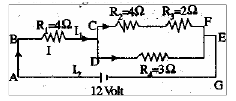B. Electric current in circuit

C. Current I1

D. Current I2

Known :

Resistor 1 (R1) = 4 Ω

Resistor 2 (R2) = 4 Ω

Resistor 3 (R3) = 2 Ω

Resistor 4 (R4) = 3 Ω

Electric voltage (V) = 12 Volt

Solution :

A. Total resistance (R)

Resistor R2 and resistor R3 are connected in series. The equivalent resistor :

R23 = R2 + R3 = 4 Ω + 2 Ω = 6 Ω

Resistor R23 and resistor R4 are connected in parallel. The equivalent resistor :

1/R234 = 1/R23 + 1/R4 = 1/6 + 1/3 = 1/6 + 2/6 = 3/6

R234 = 6/3 = 2 Ω

Resistor R1 and resistor R234 are connected series. The equivalent resistor :

R = R1 + R234 = 4 Ω + 2 Ω = 6 Ω

The total resistance is 6 Ohm.

B. Electric current in circuit (I)

V = I R

V = electric voltage, I = electric current, R = electric resistance

Electric current :

I = V / R = 12 Volt / 6 Ohm = 2 Ampere

C. Electric current I1

Electric current in resistor R1 = electric current in circuit = 2 Ampere.

D. Current I2

Resistor R23 and resistor R4 are connected in parallel. The equivalent resistor R234 = 2 Ohm.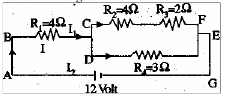Electric current in resistor R234 = electric current in resistor R1 = 2 Ampere.

Voltage in resistor R234 is:

V = I R234 = (2 A)(2 Ohm) = 4 Volt

Voltage in resistor R234 = voltage in resistor R4 = voltage in resistor R23 = 4 Volt.

The equivalent resistor R23 is 6 Ohm.

Electric current in resistor R23 is :

I = V / R = 4 Volt / 6 Ohm = 2/3 Ampere

Electric current in resistor R23 = Electric current in resistor R2 = electric current in resistor R3 = 2/3 Ampere.

[irp]

6. R1 = R2 = 10 Ω and R3 = R4 = 8 Ω. What is the electric current in circuit as shown in figure below ?

Known :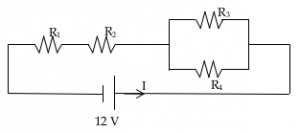Resistor R1 = Resistor R2 = 10 Ω

Resistor R3 = Resistor R4 = 8 Ω

Electric voltage (V) = 12 Volt

Wanted : electric current (I)

Solution :

The equivalent resistor

Resistor R3 and resistor R4 are connected in parallel, the equivalent resistor :

1/R34 = 1/R3 + 1/R4 = 1/8 + 1/8 = 2/8

R34 = 8/2 = 4 Ω

Resistor R1, R2 and R34 are connected in series, the equivalent resistor :

R = R1 + R2 + R34 = 10 Ω + 10 Ω + 4 Ω = 24 Ω

Electric current :

I = V / R = 12 Volt / 24 Ohm = 0,5 Volt/Ohm = 0.5 Ampere

[irp]

7. If the internal resistance of battery ignored, what is the electric current in the circuit shown in figure below.

Known :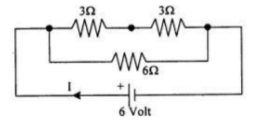Resistor R1 = 3 Ohm

Resistor R2 = 3 Ohm

Resistor R3 = 6 Ohm

Electric voltage (V) = 6 Volt

Wanted : Electric current (I)

Solution :

Equivalent resistor

Resistor R1 and R2 are connected in series. The equivalent resistor :

R12 = R1 + R2 = 3 Ohm + 3 Ohm = 6 Ohm

Resistor R12 and resistor 3 are connected in parallel. The equivalent resistor :

1/R = 1/R12 + 1/R3 = 1/6 + 1/6 = 2/6

R = 6/2 = 3 Ohm

Electric current :

I = V / R = 6 / 3 = 2 Ampere

8. What is the total electric current in circuit as shown in figure below.

Known :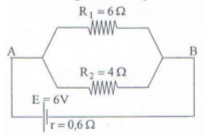Resistor R1 = 6 Ohm

Resistor R2 = 4 Ohm

Electric current (V) = 6 Volt

Internal resistance (r) = 0.6 Ohm

Wanted : Electric current

Solution :

Resistor R1 and resistor R2 are connected in parallel. The equivalent resistor :

1/RP = 1/R1 + 1/R2 = 1/6 + 1/4 = 4/24 + 6/24 = 10/24

RP = 24/10 = 2.4 Ohm

Resistor RP and internal resistance (r) are connected in series. The equivalent resistor :

R = RP + r = 2.4 Ohm + 0.6 Ohm = 3.0 Ohm

Electric current in circuit :

I = V / R = 6 Volt / 3 Ohm = 2 Ampere